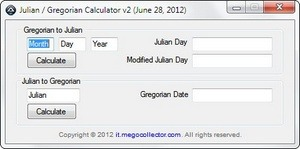# A Julian and Gregorian Calculator using AutoIt

I know that there is a Julian Day Converter that has been around for years. It, however, is no longer supported. The window can sometimes be a little messy to re-size and it is expressed by dd/mm/yyyy not mm/dd/yyyy for which I am more used to. Well, here is my attempt at a calculator using AutoIt and Koda.

This calculator calculates the Gregorian calendar for all AD dates to Julian and Modified Julian Days. It also calculates Julian to Gregorian. I have verified the dates as far back as 1/1/1500 and they check out against the references provided in the code and in a reference provided at the bottom of this article, totaling over three sources. Interestingly, the existing Julian Day Calculator differs in results by 10 days and are confirmed against U.S. Naval Observatory (“USNO”).To use, type in the month, day, and year and press calculate; or type the Julian day and press calculate to calculate the Gregorian date.

```; Sources / Resources
; http://csc.sesi-va.com/viewtopic.php?f=11&t=540
; http://scienceworld.wolfram.com/astronomy/JulianDate.html
; http://www.autoitscript.com/autoit3/docs/functions/Int.htm
; http://pmyers.pcug.org.au/General/JulianDates.htm

#include <ButtonConstants.au3>
#include <EditConstants.au3>
#include <GUIConstantsEx.au3>
#include <StaticConstants.au3>
#include <WindowsConstants.au3>

#Region ### START Koda GUI section ### Form=
\$Form1 = GUICreate("Julian / Gregorian Calculator v2 (June 28, 2012)", 434, 186, 231, 126)
\$Month = GUICtrlCreateInput("Month", 32, 26, 41, 21)
\$Day = GUICtrlCreateInput("Day", 80, 26, 41, 21)
\$Year = GUICtrlCreateInput("Year", 128, 26, 41, 21)
\$Julian_Day = GUICtrlCreateInput("", 280, 26, 121, 21)
\$Modified_Julian_Day = GUICtrlCreateInput("", 280, 51, 121, 21)
\$greg_Day = GUICtrlCreateInput("", 280, 103, 121, 21)
\$jd_input = GUICtrlCreateInput("Julian", 32,103,80,21)
\$Calculate = GUICtrlCreateButton("Calculate", 32, 48, 75, 25, 0)
\$greg_calc = GUICtrlCreateButton("Calculate", 32, 125, 75, 25, 0)
\$Label1 = GUICtrlCreateLabel("Julian Day", 224, 26, 53, 17)
\$Label2 = GUICtrlCreateLabel("Modified Julian Day", 182, 53, 96, 17)
\$Group1 = GUICtrlCreateGroup(" Gregorian to Julian ", 16, 8, 401, 75)
GUICtrlCreateGroup("", -99, -99, 1, 1)
\$Group2 = GUICtrlCreateGroup("Julian to Gregorian", 16, 85, 401, 75)
\$Label0 = GUICtrlCreateLabel("Gregorian Date", 203, 105, 77, 17)
GUICtrlCreateGroup("", -99, -99, 1, 1)
GUICtrlSetFont(-1, 8, 400, 0, "Arial")
GUICtrlSetColor(-1, 0x646464)
\$Label4 = GUICtrlCreateLabel('it.megocollector.com',  165, 166, 100, 17)
GUICtrlSetCursor(-1, 0)
GUICtrlSetColor(-1, 0x00000FF)
GUICtrlSetFont(-1, 8, 400, 0, "Arial")
GUICtrlSetTip(-1, 'https://it.megocollector.com')
GUICtrlSetFont(-1, 8, 400, 0, "Arial")
GUICtrlSetColor(-1, 0x646464)

GUISetState(@SW_SHOW)
#EndRegion ### END Koda GUI section ###

While 1
\$nMsg = GUIGetMsg()
Switch \$nMsg
Case \$GUI_EVENT_CLOSE
Exit

Case \$Calculate
Case ''
Case Else
EndSwitch

Case ''
Case Else
EndSwitch

Case ''
Case Else
EndSwitch

; For all AD dates in the Gregorian calendar.
\$mjd = (\$jd - 2400001) ;mjd = jd - 2400001

GUICtrlSetData(6, string(\$jd))
GUICtrlSetData(7, string(\$mjd))

Case \$greg_calc
Case ''
Case Else
EndSwitch

\$y = Int((4 * \$j - 1) / 146097)
\$j = 4 * \$j - 1 - 146097 * \$y
\$d = Int(\$j / 4)
\$j = Int((4 * \$d + 3) / 1461)
\$d = 4 * \$d + 3 - 1461 * \$j
\$d = Int((\$d + 4) / 4)
\$m = Int((5 * \$d - 3) / 153)
\$d = 5 * \$d - 3 - 153 * \$m
\$d = Int((\$d + 5) / 5)
\$y = 100 * \$y + \$j

If \$m < 10 Then
\$m = \$m + 3
Else
\$m = \$m - 9
\$y = \$y + 1
EndIf

\$gstring = \$m&"/"&\$d&"/"&\$y

GUICtrlSetData(8, string(\$gstring))

Case \$label4
ShellExecute("https://it.megocollector.com")

EndSwitch
WEnd```

Sources Referenced in the code.
http://www.julian-date.com/